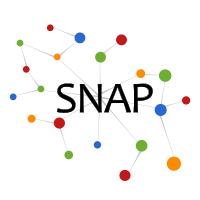# GNN-Explainer: Generating Explanations for Graph Neural Networks

GNN-Explainer is the first general tool for explaining predictions made by graph neural networks (GNNs). Given a trained GNN model and an instance as its input, the GNN-Explainer produces an explanation of the GNN model prediction via a compact subgraph structure, as well as a set of feature dimensions important for its prediction.

## Motivation

Graph Neural Networks (GNNs) are a powerful tool for machine learning on graphs. GNNs combine node feature information with the graph structure by recursively passing neural messages along edges of the input graph. However, incorporating both graph structure and feature information leads to complex models and explaining predictions made by GNNs remains unsolved.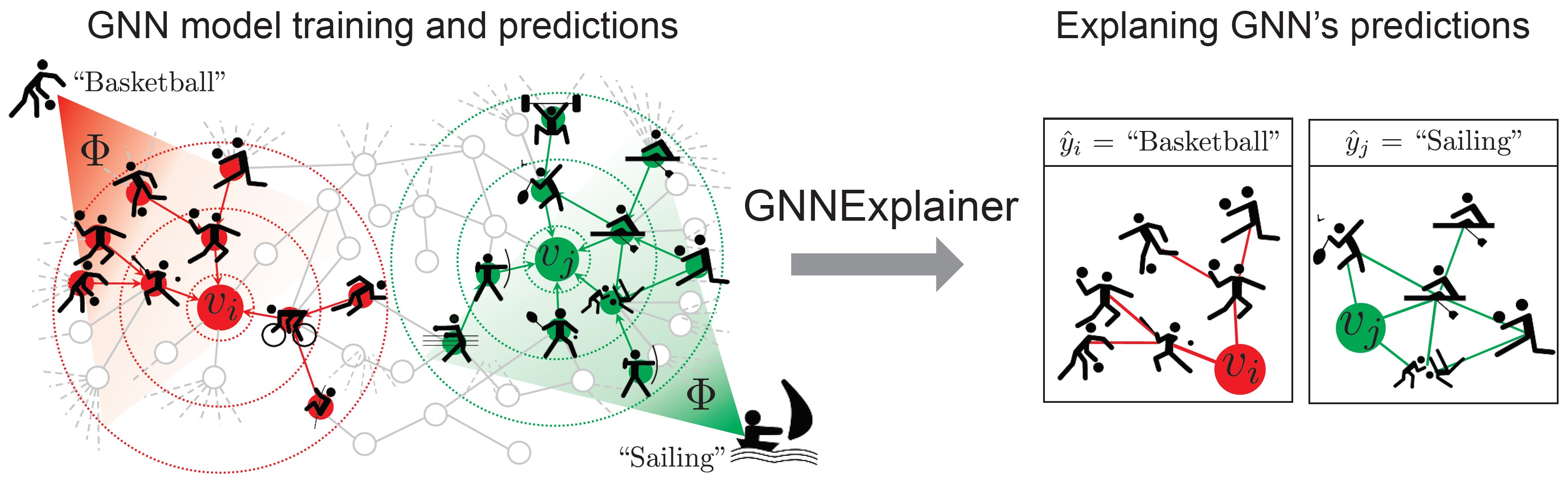GNN-Explainer is task agnostic: it can be applied to

• Node classification
• Graph classification

GNN-Explainer can be applied to many common GNN models: GCN, GraphSAGE, GAT, SGC, hypergraph convolutional networks etc.

## Method

GNN-Explainer specifies an explanation as a rich subgraph of the entire graph the GNN was trained on, such that the subgraph maximizes the mutual information with GNN’s prediction(s). This is achieved by formulating a mean field variational approximation and learning a real-valued graph mask which selects the important subgraph of the GNN’s computation graph. Simultaneously, GNN-Explainer also learns a feature mask that masks out unimportant node features.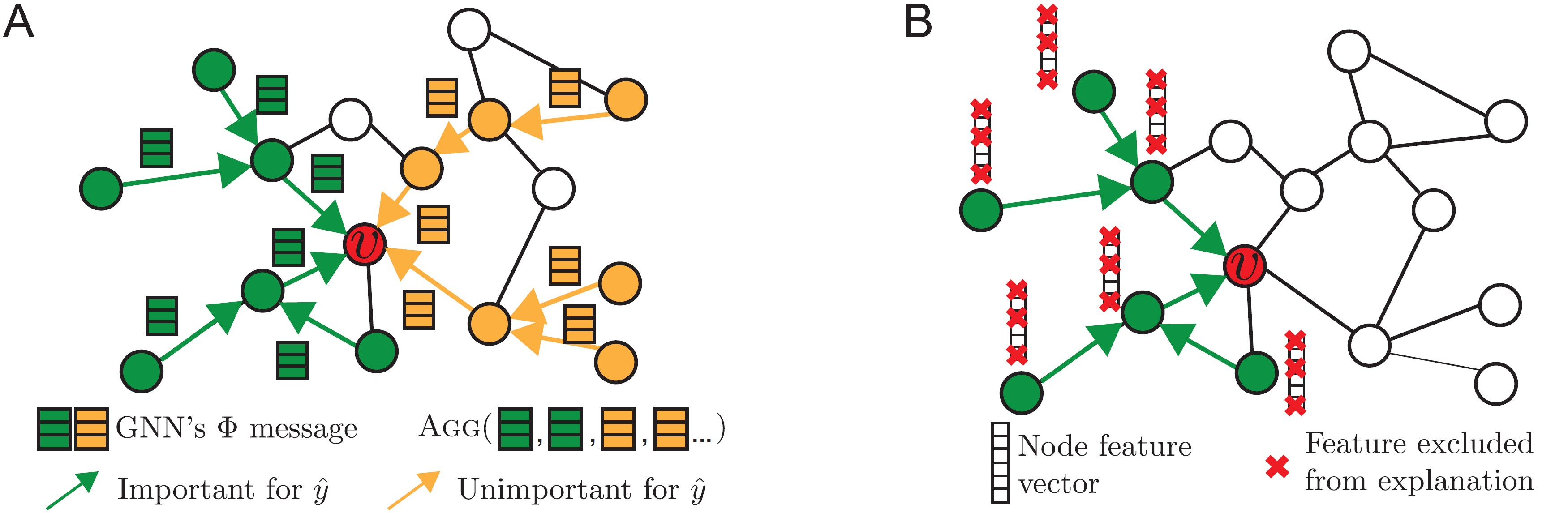In the above figure, for a prediction on the red node (node classification), an important subgraph structure (in green) is identified (left). The green edges form important neural message-passing pathways, which allow useful node information to be propagated across the computation graph (node neighborhood) and aggregated at v for prediction, while other edges do not (orange). In addition, GNN-Explainer also identifies what feature dimensions of the subgraph's nodes are important for prediction (right).

GNN-Explainer achieves this task via optimization: it tries to learn a mask on the adjacency matrix of the computation graph, and a feature mask, to minimize the uncertainty of the prediction made by the model (minimize conditional entropy conditioned on important subgraph and feature dimensions). Furthermore, we treat explanation (subgraph + feature dimensions) as being drawn from a distribution of "plausible explanations", so that we can perform continuous relaxation which makes gradient descent on masks computationally feasible.

Lastly we add regularizations on size of the subgraph and number of selected feature dimensions, to enforce the conciseness of the explanation.

We evaluate on synthetic node classification datasets, since we can construct the datasets such that we know the groundtruth explanation. They are illustrated in the following figure. The basis graph is either a Barabasi-Albert random graph, or a tree (256 to 512 nodes). We then randomly attach 80 motif structures (in green) to the basis graph. Node labels are the nodes' roles (whether they are in basis graph, or one of the roles/orbits in the motif).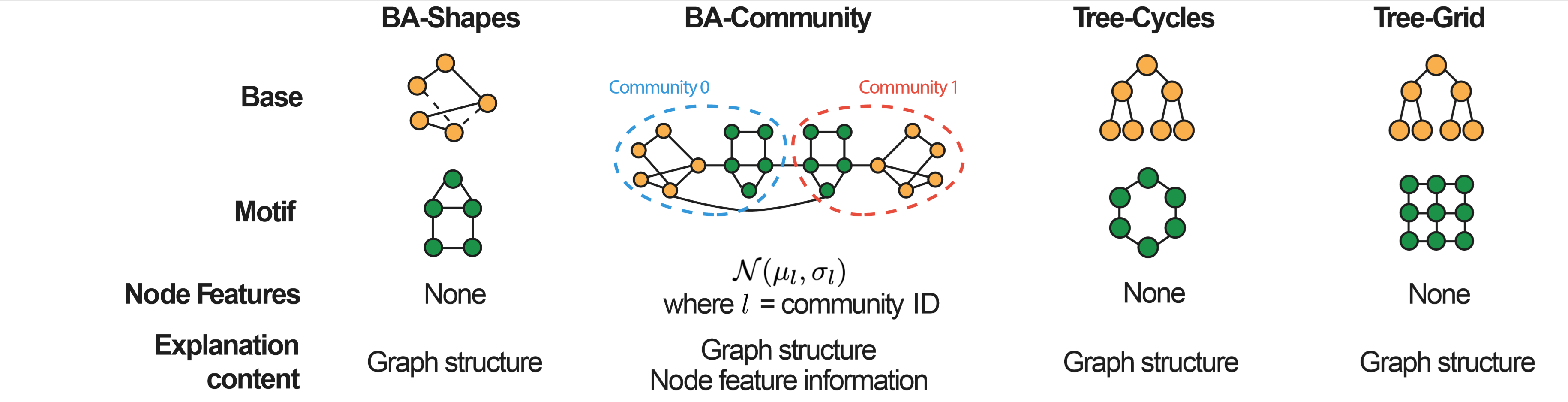We compare our explanation with possible alternative approaches (based on gradients and graph attention).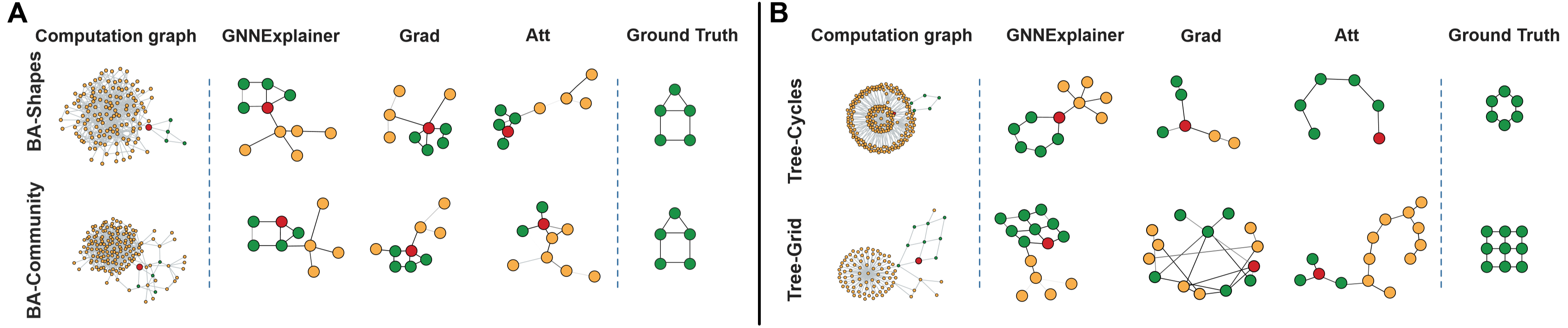We further test GNN-Explainer on real graph classification benchmarks: Mutagenicity and Reddit-Binary, observing interesting patterns in explanation. Mutagenic molecules tend to have rings and NO2 or NH2 functional groups. In Reddit community graph, QA community tends to have 2 or 3 center nodes that are both connected to many nodes of degree 2.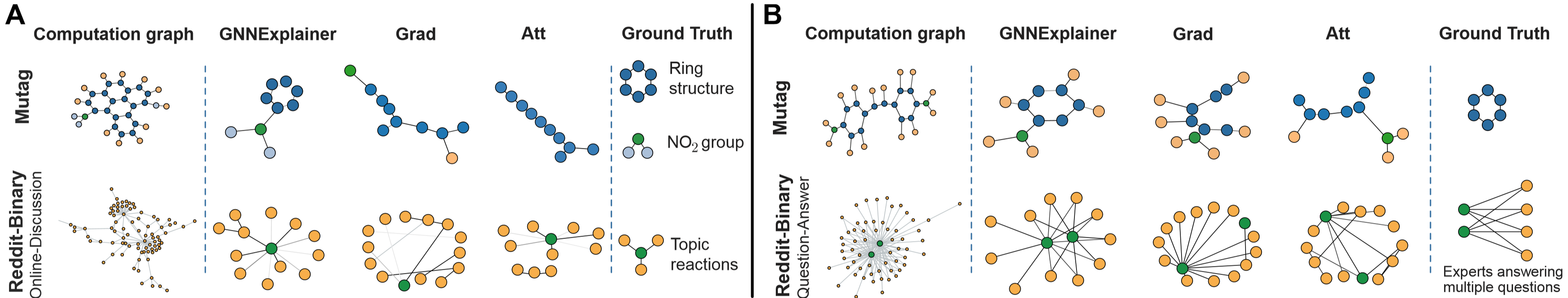Finally we note that the explanation size can be tuned with regularization strength: the larger the regularization constant, the more concise explanation that the model tends to produce.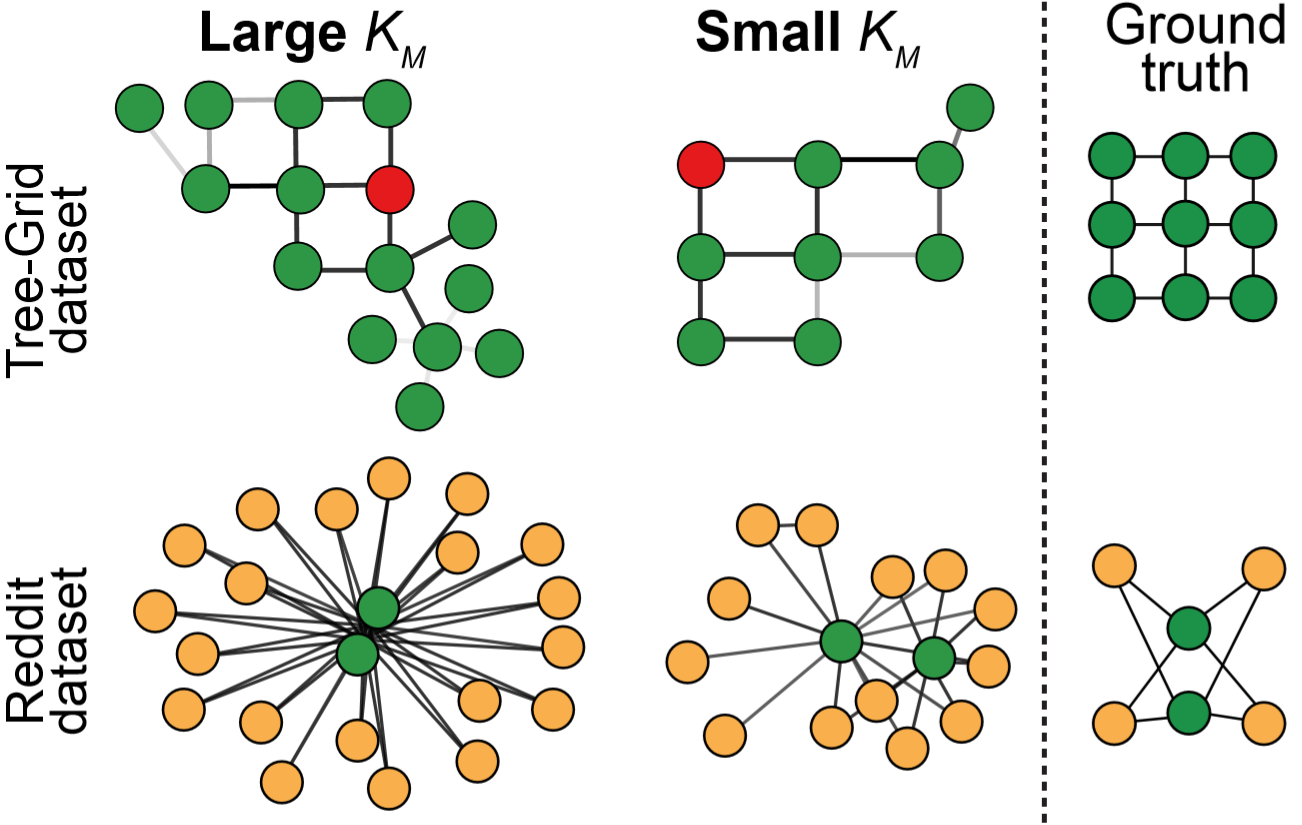Note: in some situations where masking can cause a flipping of label, we can modify the objective so that instead of optimizing the conditional entropy (model certainty), we can just maximize the prediction probability of the correct class.

Please refer to our paper for detailed explanations and more results.

## Code

A reference implementation of GNN-Explainer and interactive notebook demo will be made public on Nov 15th 2019.

## Datasets

Mutagenicity and Reddit-Binary datasets can be found here.

## Contributors

The following people contributed to GNN-Explainer:
Rex Ying
Dylan Bourgeois
Jiaxuan You
Marinka Zitnik
Jure Leskovec

## References

GNNExplainer: Generating Explanations for Graph Neural Networks, . R. Ying, D. Bourgeois, J. You, M. Zitnik, J. Leskovec. Neural Information Processing Systems (NeurIPS), 2019.